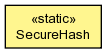## org.jboss.dna.common.util Class SecureHash

```java.lang.Objectorg.jboss.dna.common.util.SecureHash
```

```@Immutable
public class SecureHashextends Object```A simple utility to generate various kinds of secure hashes.

Nested Class Summary
`static class` `SecureHash.Algorithm`
Commonly-used hashing algorithms.

Constructor Summary
`SecureHash()`

Method Summary
`static byte[]` ```getHash(SecureHash.Algorithm algorithm, byte[] content)```
Get the hash of the supplied content, using the supplied digest algorithm.
`static byte[]` ```getHash(SecureHash.Algorithm algorithm, File file)```
Get the hash of the supplied content, using the supplied digest algorithm.
`static byte[]` ```getHash(SecureHash.Algorithm algorithm, InputStream stream)```
Get the hash of the supplied content, using the supplied digest algorithm.
`static byte[]` ```getHash(String digestName, byte[] content)```
Get the hash of the supplied content, using the digest identified by the supplied name.
`static byte[]` ```getHash(String digestName, File file)```
Get the hash of the supplied content, using the digest identified by the supplied name.
`static byte[]` ```getHash(String digestName, InputStream stream)```
Get the hash of the supplied content, using the digest identified by the supplied name.

Methods inherited from class java.lang.Object
`equals, getClass, hashCode, notify, notifyAll, toString, wait, wait, wait`

Constructor Detail

### SecureHash

`public SecureHash()`
Method Detail

### getHash

```public static byte[] getHash(SecureHash.Algorithm algorithm,
byte[] content)
throws NoSuchAlgorithmException```
Get the hash of the supplied content, using the supplied digest algorithm.

Parameters:
`algorithm` - the hashing function algorithm that should be used
`content` - the content to be hashed; may not be null
Returns:
the hash of the contents as a byte array
Throws:
`NoSuchAlgorithmException` - if the supplied algorithm could not be found
`IllegalArgumentException` - if the algorithm is null

### getHash

```public static byte[] getHash(SecureHash.Algorithm algorithm,
File file)
throws NoSuchAlgorithmException,
IOException```
Get the hash of the supplied content, using the supplied digest algorithm.

Parameters:
`algorithm` - the hashing function algorithm that should be used
`file` - the file containing the content to be hashed; may not be null
Returns:
the hash of the contents as a byte array
Throws:
`NoSuchAlgorithmException` - if the supplied algorithm could not be found
`IllegalArgumentException` - if the algorithm is null
`IOException` - if there is an error reading the file

### getHash

```public static byte[] getHash(SecureHash.Algorithm algorithm,
InputStream stream)
throws NoSuchAlgorithmException,
IOException```
Get the hash of the supplied content, using the supplied digest algorithm.

Parameters:
`algorithm` - the hashing function algorithm that should be used
`stream` - the stream containing the content to be hashed; may not be null
Returns:
the hash of the contents as a byte array
Throws:
`NoSuchAlgorithmException` - if the supplied algorithm could not be found
`IllegalArgumentException` - if the algorithm is null
`IOException` - if there is an error reading the stream

### getHash

```public static byte[] getHash(String digestName,
byte[] content)
throws NoSuchAlgorithmException```
Get the hash of the supplied content, using the digest identified by the supplied name.

Parameters:
`digestName` - the name of the hashing function (or `message digest`) that should be used
`content` - the content to be hashed; may not be null
Returns:
the hash of the contents as a byte array
Throws:
`NoSuchAlgorithmException` - if the supplied algorithm could not be found

### getHash

```public static byte[] getHash(String digestName,
File file)
throws NoSuchAlgorithmException,
IOException```
Get the hash of the supplied content, using the digest identified by the supplied name.

Parameters:
`digestName` - the name of the hashing function (or `message digest`) that should be used
`file` - the file whose content is to be hashed; may not be null
Returns:
the hash of the contents as a byte array
Throws:
`NoSuchAlgorithmException` - if the supplied algorithm could not be found
`IOException` - if there is an error reading the file

### getHash

```public static byte[] getHash(String digestName,
InputStream stream)
throws NoSuchAlgorithmException,
IOException```
Get the hash of the supplied content, using the digest identified by the supplied name. Note that this method never closes the supplied stream.

Parameters:
`digestName` - the name of the hashing function (or `message digest`) that should be used
`stream` - the stream containing the content to be hashed; may not be null
Returns:
the hash of the contents as a byte array
Throws:
`NoSuchAlgorithmException` - if the supplied algorithm could not be found
`IOException` - if there is an error reading the stream